Next: Determination of Metacentric Height Up: Hydrostatics Previous: Vertical Stability of Floating

# Angular Stability of Floating Bodies

Let us now investigate the stability of floating bodies to angular displacements. For the sake of simplicity, we shall only consider bodies that have two mutually perpendicular planes of symmetry. Suppose that when such a body is in an equilibrium state the two symmetry planes are vertical, and correspond to theand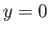planes. As before, the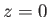plane coincides with the surface of the water. It follows, from symmetry, that when the body is in an equilibrium state its center of gravity,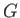, and center of buoyancy,, both lie on the-axis.Suppose that the body turns through a small angleabout some horizontal axis, lying in the plane, that passes through the origin. Let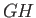be that, originally vertical, straight-line that passes through the body's center of gravity,, and original center of buoyancy,. Owing to the altered shape of the volume of displaced water, the center of buoyancy is shifted to some new position. Let the vertical straight-line passing throughmeetat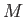. (See Figure 2.1.) Point(in the limit) is called the metacenter. In the disturbed state, the body's weight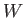acts downward through, and the buoyancy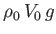acts upward through. Let us assume that the submerged volume,, is unchanged from the equilibrium state (which excludes vertical oscillations from consideration). It follows that the weight and the buoyancy force are equal and opposite, so that there is no net force on the body. However, as can be seen from Figure 2.1, the weight and the buoyancy force generate a net torque of magnitude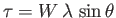. Here,is the length: that is, the distance between the metacenter and the center of gravity. This distance is generally known as the metacentric height, and is defined such that it is positive whenlies above, and vice versa. Moreover, as is also clear from Figure 2.1, whenlies abovethe torque acts to reduce, and vice versa. In the former case, the torque is known as a righting torque. We conclude that a floating body is stable to small angular displacements about some horizontal axis lying in the planeprovided that its metacentric height is positive: that is, provided that the metacenter lies above the center of gravity. Because we have already demonstrated that a floating body is unconditionally stable to small vertical displacements (and as it is also fairly obvious that such a body is neutrally stable to both horizontal displacements and angular displacements about a vertical axis passing through its center of gravity), it follows that a necessary and sufficient condition for the stability of a floating body to a general small perturbation (made up of arbitrary linear and angular components) is that its metacentric height be positive for angular displacements about any horizontal axis.Next: Determination of Metacentric Height Up: Hydrostatics Previous: Vertical Stability of Floating
Richard Fitzpatrick 2016-03-31